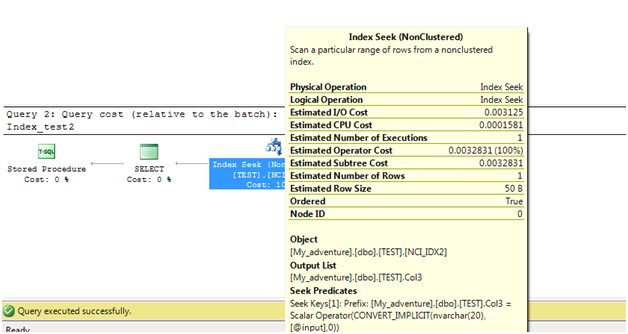### Sql Server - Various observations on indexes

In this post, let us see various observation on how the query behaves when Indexes are created. First let us create table & insert sample data.

```CREATE TABLE TEST (Col1  INT ,Col2 VARCHAR(20),Col3 NVARCHAR(20))

;WITH CTE (Col1,Col2,Col3)
AS(
SELECT Col1 = 1,Col2 = 'varchar column' + CAST(1 AS VARCHAR(20)),col3 = 'nvarchar column' + CAST(1 AS NVARCHAR(20))
UNION ALL
SELECT Col1 = Col1 + 1,Col2 = 'varchar column' + CAST(Col1 + 1 AS VARCHAR(20)),col3 = 'nvarchar column' + CAST(Col1 + 1 AS NVARCHAR(20))
FROM CTE
WHERE Col1 < 30000
)

INSERT INTO TEST
SELECT * FROM CTE OPTION (MAXRECURSION 30000)

SELECT * FROM TEST
```

Note: Press ctrl + M/ctrl + L, to see the execution plans for all below examples.

/-------------------------------------------------------------------------/

Example 1
First create non clustered index NCI_IDX1 and try the below select.

```CREATE NONCLUSTERED INDEX NCI_IDX1 ON TEST (Col2,Col3)

--query1
SELECT * FROM TEST WHERE Col2 = 'varchar column500'
```

You will notice, query1 going for index seek with RID lookup. It is because, we are selecting all columns in table, in that query Col1 is not covered under any index.

We can make the same query go for 100% index seek, by just including Col1 in
nonclustered index NCI_IDX1 like shown below.

```CREATE NONCLUSTERED INDEX NCI_IDX1 ON TEST (Col2,Col3) INCLUDE (Col1)
```

The above index with INCLUDE clause is the covering index. Covering index is basically used to cover the query(include columns from Select list which are not part of index) and to avoid bookmark lookup. 1023 columns can added in the INCLUDE clause.

Also try the steps in Example 1.1

Example 1.1

Step 1: Now drop nonclustered index NCI_IDX1

```DROP INDEX NCI_IDX1 ON TEST
```

Step 2: Create clustered index CI_IDX

```CREATE CLUSTERED INDEX CI_IDX ON TEST (Col1)
```

Step 3: Again create nonclustered index NCI_IDX1 and then try query1

```CREATE NONCLUSTERED INDEX NCI_IDX1 ON TEST (Col2,Col3)
--query1
SELECT * FROM TEST WHERE Col2 = 'varchar column500'
```

You will notice, query1 going for 100 % index seek by creating clustered indexIf your table is going to have frequent DML operations, having clustered index will be a costly operation.Because if you are inserting new records, clustered index to maintain order of data in index page, it has to insert data in particular page, consider if that page is already full, it may result in page splits. We can handle page splits, by setting appropriate fill factor.

#### Summary & Recommendations :

From Example 1 & Example 1.1

1.) If you create nonclustered indexes first and then clustered index, internally all nonclustered indexes on that table would again get recreated.
So it is always advisable to create clustered index first before creating nonclustered indexes.

2.) Also nonclustered indexes will always point to the clustered index internally.
For example:

```ALTER TABLE TEST ADD Col4 BIGINT
```

If you query the below select, without clustered index it will go for RID lookup,
With clustered index it will go for Key lookup using clustered index key.

```SELECT Col2,Col4 FROM TEST WHERE Col2 = 'varchar column500'
```

/-------------------------------------------------------------------------/

Example 2

Step 1:

```DROP INDEX NCI_IDX1 ON TEST
```

Step 2:

```CREATE NONCLUSTERED INDEX NCI_IDX2 ON TEST (Col3,Col2)
```

Step 3:

```--Query1 :
SELECT Col2 FROM TEST WHERE Col2 = 'varchar column500'
--Query2 :
SELECT Col3 FROM TEST WHERE Col3 = 'nvarchar column500'
```

You can notice that, though index is created on Col3 & Col2, Query 1 going for scan and Query 2 going for seek. Now try below steps

Step 4:

```DROP INDEX NCI_IDX2 ON TEST
```

Step 5:

```CREATE NONCLUSTERED INDEX NCI_IDX1 ON TEST (Col2,Col3)
```

Step 6:

```--Query1 :
SELECT Col2 FROM TEST WHERE Col2 = 'varchar column500'
--Query2 :
SELECT Col3 FROM TEST WHERE Col3 = 'nvarchar column500'
```

You can notice that, though index is created on Col2 & Col3, Query 1 going for seek and Query 2 going for scan.

#### Summary & Recommendations :

From Example 2
1.) Care should be taken in the order of columns while creating index, to make full use of index .

/-------------------------------------------------------------------------/

Example 3.1

Step 1:

```CREATE PROCEDURE Index_test1 (@input VARCHAR(20))
AS
BEGIN
SELECT Col2 FROM TEST WHERE Col2 = @input
END
--DBCC DROPCLEANBUFFERS
```

Step 2:

```EXEC Index_test1 'varchar column500'
```

You will notice, execution of procedure going for seek. This is because, procedure input parameter data type (VARCHAR) & column data type (VARCHAR) are same.

Step 3:

Alter the procedure, by changing @input VARCHAR(20) to @input NVARCHAR(20)

Step 4: Again execute

```EXEC Index_test1 'varchar column500'
```

You will notice,execution of procedure going for scan.This is because,you can notice in below image, under predicate column data type VARCHAR of lower precedence has been converted to procedure input parameter data type NVARCHAR of higher precedence.

Example 3.2

```--Step 1 :
DROP INDEX NCI_IDX1 ON TEST

--Step 2:
CREATE NONCLUSTERED INDEX NCI_IDX2 ON TEST (Col3,Col2)

--Step 3:
CREATE PROCEDURE Index_test2 (@input NVARCHAR(20))
AS
BEGIN
SELECT Col3 FROM TEST WHERE Col3 = @input
END

--Step 4:
--DBCC DROPCLEANBUFFERS
EXEC Index_test2 'nvarchar column500'
```

You will notice, execution of procedure going for seek. This is because, procedure input parameter data type (NVARCHAR) & column data type (NVARCHAR) are same.

Step 5: Alter the procedure, by changing @input NVARCHAR(20) to @input VARCHAR(20)

Step 6: Again execute

```EXEC Index_test2 'nvarchar column500'
```

You will notice, execution of procedure again going for seek. Though input parameter data type (VARCHAR) & column data type (NVARCHAR) are different. This is because, you can notice in below image,under predicate it has converted data type of procedure input parameter not the data type of columnYou can refer this site for sql server data type precedence chart.

#### Summary & Recommendations :

From Example 3.1 & Example 3.2

1.) Care should be taken while declaring data type inside procedures, to avoid unnecessary performance degradation because of data type mismatch.

--DROP TABLE TEST